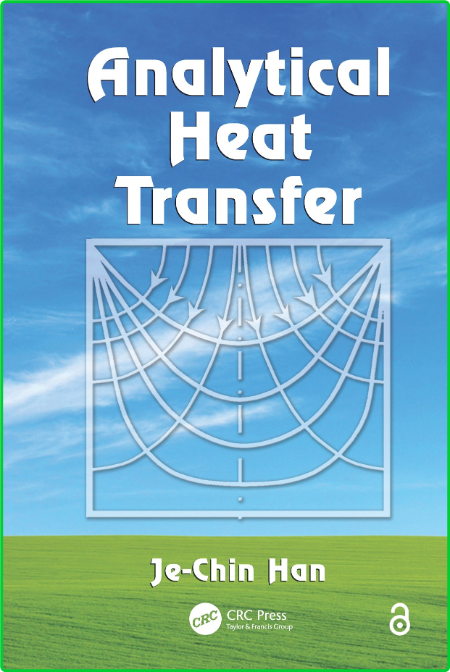# Analytical Heat Transfer

Posted in: eBooks | By: Jocker | 19-07-2021, 12:56 | 0 Comments
19
July
2021Analytical Heat Transfer (True PDF)
pdf | 11.27 MB | English | Isbn:‎ 978-0367382544 | Author: Je-Chin Han | Year: 2019

Description:

Developed from the author's 30 years of teaching a graduate-level intermediate heat transfer course, Analytical Heat Transfer explains how to analyze and solve conduction, convection, and radiation heat transfer problems. Suitable for entry-level graduate students, the book fills the gap between basic heat transfer undergraduate courses and advanced heat transfer graduate courses.

The author places emphasis on modeling and solving engineering heat transfer problems analytically, rather than simply applying the equations and correlations for engineering problem calculations. He describes many well-known analytical methods and their solutions, such as Bessel functions, separation of variables, similarity method, integral method, and matrix inversion method. He also presents step-by-step mathematical formula derivations, analytical solution procedures, and numerous demonstration examples of heat transfer applications.

By providing a strong analytical background, the text enables students to tackle complex engineering heat transfer problems encountered in practice. This analytical knowledge also helps them to read and understand heat transfer-related research papers.

Category:Thermodynamics, Mechanics, Civil Engineering